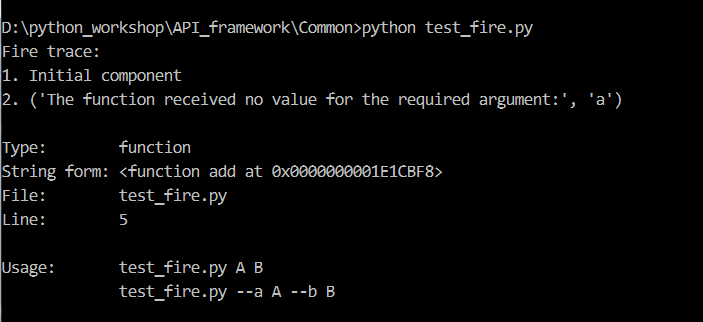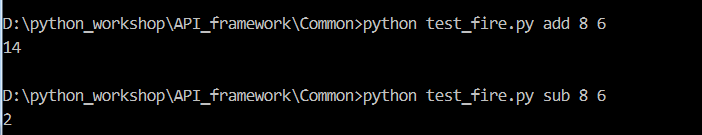# python fire 使用教程

### 一. 介绍

fire是python中用于生成命令行界面(Command Line Interfaces, CLIs)的工具，不需要做任何额外的工作，只需要从主模块中调用fire.Fire()，它会自动将你的代码转化为CLI，Fire()的参数可以说任何的python对象

### 二. 安装

``````pip install fire
``````

### 三. 用法

``````import fire

count = a + b
return count

if __name__ == '__main__':
````````````import fire

count = a + b
return count

def sub(a, b):
result = a - b
return result

if __name__ == '__main__':
fire.Fire()
````````````import fire

class Calculator(object):
count = a + b
return count

def sub(self, a, b):
result = a - b
return result
# Python学习交流群：711312441
if __name__ == '__main__':
fire.Fire(Calculator)    #这里用类名Calculator或者类的实例化对象Calculator()结果都是一样的
``````